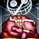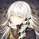1001 просмотров
Linearly combining moving averages can provide relatively interesting results such as a low-lagging moving averages or moving averages able to produce more pertinent crosses with the price.

As a remainder, a linear combination is a mathematical expression that is based on the multiplication of two variables (or terms) with two coefficients (also called scalars when working with vectors) and adding the results, that is:

ax + by

This expression is a linear combination , with x/y as variables and a/b as coefficients. Lot of indicators are made from linear combinations of moving averages, some examples include the double/ triple exponential moving average , least squares moving average and the hull moving average .

Today proposed indicator allow the user to combine many types of moving averages together in order to get different results, we will introduce each settings of the indicator as well as how they affect the final output.

Explaining The Effects Of Linear Combinations

There are various ways to explain why linear combination can produce low-lagging moving averages, lets take for example the linear combination of a fast SMA of period p/2 and slow simple moving average of period p, the linear combination of these two moving averages is described as follows:

MA = 2SMA(p/2) + -1SMA(p)

Which is equivalent to:

MA = 2SMA(p/2) - SMA (p) = SMA (p/2) + SMA (p/2) - SMA (p)

We can see the above linear combinations consist in adding a bandpass filter to the fast moving average, which of course allow to reduce the lag. It is important to note that lag is reduced when the first moving average term is more reactive than the second moving average term. In case we instead use:

MA = -2SMA(p/2) + 1SMA(p)

we would have a combination between a low-pass and band-reject filter.

The Indicator

The indicator is based on the following linear combination:

Coeff × LeadingMA(length) - (Coeff-1) × LaggingMA(length)

The length setting control both moving averages period, leading control the type of moving average used as leading MA, while lagging control the type of MA used as lagging moving average, in order to get low lag results the leading MA should be more reactive than the lagging MA. Coeff control the coefficients of the linear combination, with higher values of coeff amplifying the effects of the linear combination, negative values of coeff would make a low-lag moving average become a lagging moving average, coeff = 1 return the leading MA, coeff = -1 return the lagging MA. The leading period divisor allow to divide the period of the leading MA by the selected number.

The types of moving average available are: simple, exponentially weighted, triangular, least squares, hull and volume weighted. The lagging MA allow you to select another MA on the chart as input.

length = 100, leading period divisor = 2, coeff = 2, with both MA type = SMA . Using coeff = -2 instead would give:

You can select "Plot leading and lagging" in order to show the leading and lagging MA.

Conclusion

The proposed tool allow the user to create a custom moving averages by making use of linear combination. The script is not that useful when you think about it, and might maybe be one of my worst, as it is relatively impractical, not proud of it, but it still took time to make so i decided to post it anyway.

« Je suis las des cruautés de mes semblables, qui ne sont pas mes pareils.

« Je prendrai l’essor et je m’envolerai vers la mer.

« Je connaîtrai le goût des brises du large. J’entendrai les grands cris de la tempête.

## Комментарииlooks good! I have no experience what so ever with coding. but I might have a cool idea if its even possible of course (haven't been able to find an indicator yet that does this) But do you think its possible for an indicator to spot market structure and give signals on breaks and retests for example? maybe with correlation key levels of higher timeframes idk hahaha :)
ОтветитьOh, For a change longer script haha

Thank you, will check it out :)
ОтветитьOwl
@Owl, Thx, i believe the scripts that i recently posted have all more than 20 lines ^^
Ответить